#### You may also like### Is There a Theorem?

Draw a square. A second square of the same size slides around the first always maintaining contact and keeping the same orientation. How far does the dot travel?### Coins on a Plate

Points A, B and C are the centres of three circles, each one of which touches the other two. Prove that the perimeter of the triangle ABC is equal to the diameter of the largest circle.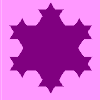### Smaller and Smaller

Can you predict, without drawing, what the perimeter of the next shape in this pattern will be if we continue drawing them in the same way?

# On the Edge

##### Age 11 to 14 Challenge Level:

Here are four tiles: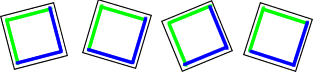They can be arranged in a $2$ by $2$ square so that this large square has a green edge: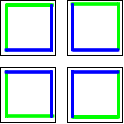If the tiles are moved around, we can make a $2$ by $2$ square with a blue edge: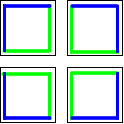If I had nine tiles it would be quite easy to paint them so that, when they were arranged in a $3$ by $3$ square, the edge of this large square is green. I would need four tiles for the corners of the square, four tiles for the edges and one tile would go in the middle of the square so wouldn't need painting at all.

This is how the green-edged square would be made: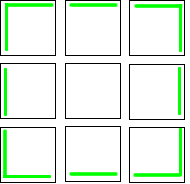But I also want to be able to make a square with a blue edge and another square with a yellow edge.

How can the other sides of these tiles be painted so that all nine tiles can be rearranged to make two more $3$ by $3$ squares - one with a blue edge and one with a yellow edge?

Now try to colour sixteen tiles so that four $4$ by $4$ squares can be made - one with a green edge, one with a blue edge, one with a yellow edge and one with a red edge.

Find a way to colour $25$ tiles so that five $5$ by $5$ squares can be made, each with a differently coloured edge.

Do you think this is possible for $36$ tiles and six coloured edges?

Will it always be possible to add an extra colour as the squares get larger?

For a 3D version of this problem why not try " Inside Out "?